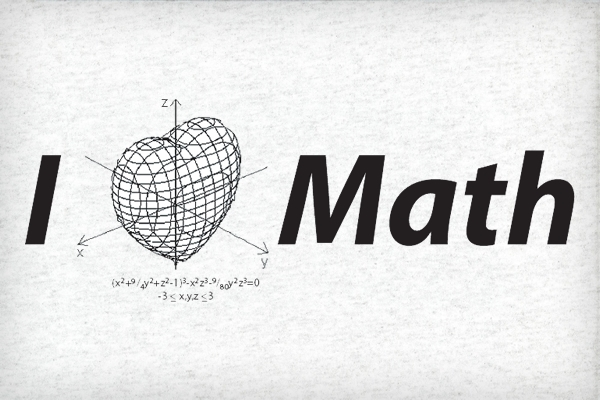The Collatz Conjecture is one of the Unsolved problems in mathematics, especially in Number Theory. The Collatz Conjecture is also termed as 3n+1 conjecture, Ulam Conjecture, Kakutani’s Problem, Thwaites Conjecture, Hasse’s Algorithm, Syracuse Problem.

Statement:

Halve it, if it is even.
Or
triple it and add 1, if it is odd.

If you keep repeating this procedure, you shall reach the number 1 at last.

Illustrations

» Starting with 1 — we get 1 in first step.
» Starting with 2 (even) — we get 1 in second step and in one operation $2 \to 1$
» Starting with 3 (odd) — we get 1 in 8th step $3 \to 10 \to 5 \to 16 \to 8 \to 4 \to 2 \to 1$

Similarly, you can check this conjecture for every positive integer; you should get 1 at last according to this conjecture.

Mathematical Illustration

Let $\mathbf {n}$ be a positive integer. Then it either be even or odd.
A. If n is even: Divide $\mathbf {n}$ by $\mathbf {2}$ and get $\mathbf {\frac {n}{2} }$ . Is it 1? — conjecture applies on that positive integer. Again if it is even — redo the same work. If it is odd, then— see next step!
B. If n is odd: Multiply $\mathbf n$ by $\mathbf 3$ & then add $\mathbf 1$ to find $\mathbf 3n+1$ . Is it 1? — conjecture applies on that positive integer. Again if it is even — redo the same work you did in A. If it is odd, then— redo the work of B!

Problem in this Conjecture

This conjecture has been tried on various kind of numbers, and those numbers have satisfied the Collatz Conjecture. But the question is that —

Is this conjecture applicable to every positive integer?

Note

Mathematicians have found no good use of Collatz Conjecture in Mathematics, so it is considered as a useless conjecture. But overall, it is unsolved — and we can’t leave any unknown or unsolved problems & principles in Math.

Feel free to ask questions, send feedback and even point out mistakes. Great conversations start with just a single word. How to write better comments?
1.to.ly-ADU5 says:

Hey there! Quick question that’s totally off topic.

Do you know how to make your site mobile friendly?
My web site looks weird when viewing from my iPhone.
I’m trying to find a theme or plugin that might be able to correct this issue.
If you have any recommendations, please share.

Appreciate it!

2.shubham choudhary says:

I can solve collatz problem

This site uses Akismet to reduce spam. Learn how your comment data is processed.

Largest Prime Numbers

What is a Prime Number? An integer, say $p$ , [ $\ne {0}$ & $\ne { \pm{1}}$ ] is said to be a prime integer iff its only factors (or divisors) are $\pm{1}$ & $\pm{p}$ . As? Few easy examples are: $\pm{2}, \pm{3}, \pm{5}, \pm{7}, \pm{11}, \pm{13}$ …….etc.…Complete Elementary Analysis of Nested radicals

This is a continuation of the series of summer projects sponsored by department of science and technology, government of India. In this project work, I have worked to collect and expand what Ramanujan did with Nested Radicals and summarized all important facts into the one article. In the article, there are formulas, formulas and only formulas — I think this…

Two Interesting Math Problems

Problem1: Smallest Autobiographical Number: A number with ten digits or less is called autobiographical if its first digit (from the left) indicates the number of zeros it contains,the second digit the number of ones, third digit number of twos and so on. For example: 42101000 is autobiographical. Find, with explanation, the smallest autobiographical number. Solution of Problem 1 Problem 2:…A Possible Proof of Collatz Conjecture

Our reader Eswar Chellappa has sent his work on the solution of ‘3X+1’ problem, also called Collatz Conjecture. He had been working on the proof of Collatz Conjecture off and on for almost ten years. The Collatz Conjecture can be quoted as follow: Let $\phi : \mathbb{N} \to \mathbb{N}^+$ be a function defined  such that: \phi(x):= \begin{cases} \frac{x}{2}, & \text{if }…

Interesting Egyptian Fraction Problem

Here is an interesting mathematical puzzle alike problem involving the use of Egyptian fractions, whose solution sufficiently uses the basic algebra. Problem Let a, b, c, d and e be five non-zero complex numbers, and; $a + b + c + d + e = -1$ … (i) $a^2+b^2+c^2+d^2+e^2=15$ …(ii) $\dfrac{1}{a} + \dfrac{1}{b} +\dfrac{1}{c} +\dfrac{1}{d} +\dfrac{1}{e}= -1$…